#Factoring and Roots of Polynomials#### What is factoring?

If you write a polynomial as the product of two or more polynomials, you have factored the polynomial. Here is an example: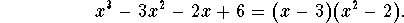The polynomials x-3 and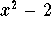are called factors of the polynomial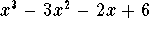. Note that the degrees of the factors, 1 and 2, respectively, add up to the degree 3 of the polynomial we started with. Thus factoring breaks up a complicated polynomial into easier, lower degree pieces.

We are not completely done; we can do better: we can factor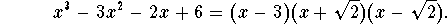We have now factored the polynomial into three linear (=degree 1) polynomials. Linear polynomials are the easiest polynomials. We can't do any better. Whenever we cannot factor any further, we say we have factored the polynomial completely.

#### Roots of polynomials.

An intimately related concept is that of a root, also called a zero, of a polynomial. A number x=a is called a root of the polynomial f(x), if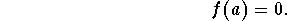Once again consider the polynomial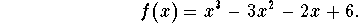Let's plug in x=3 into the polynomial.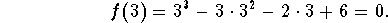Consequently x=3 is a root of the polynomial. Note that (x-3) is a factor of.

Let's plug in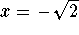into the polynomial: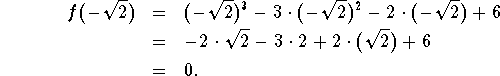Thus,is a root of the polynomial. Note that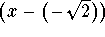is a factor of.

#### Roots and factoring.

This is no coincidence! When an expression (x-a) is a factor of a polynomial f(x), then f(a)=0.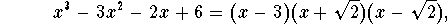there is an easier way to check that x=3 andare roots of f(x), using the right-hand side: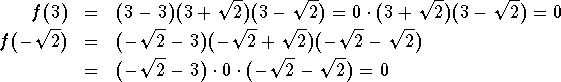Does this work the other way round? Let's look at an example: consider the polynomial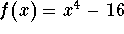. Note that x=2 is a root of f(x), since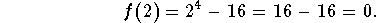Is (x-2) a factor of? You bet! We can check this by using long polynomial division: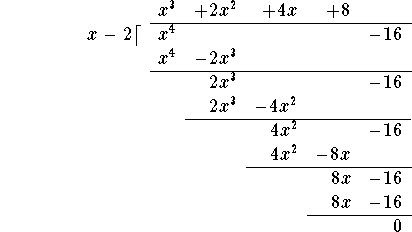So we can factor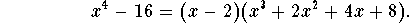Let's sum up: Finding a root x=a of a polynomial f(x) is the same as having (x-a) as a linear factor of f(x). More precisely:

Given a polynomial f(x) of degree n, and a number a, then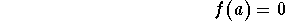if and only if there is a polynomial q(x) of degree n-1 so that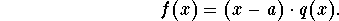#### Exercise 1.

Write down a polynomial with roots x=1, x=2, and x=3/4.

#### Exercise 2.

Write down a polynomial with roots x=-1, x=3, and x=0.

#### Exercise 3.

Factor the polynomial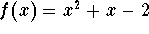. Note that f(-2)=0.

#### Exercise 4.

Factor the polynomial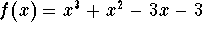completely. Note that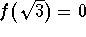and.[Back] [Next]
[Algebra] [Trigonometry] [Complex Variables]
[Calculus] [Differential Equations] [Matrix Algebra]S.O.S MATHematics home page

Do you need more help? Please post your question on our S.O.S. Mathematics CyberBoard.Helmut Knaust
Sun Jun 8 13:42:54 MDT 1997